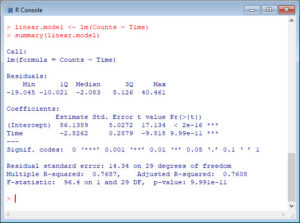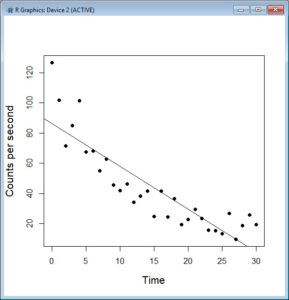In Part 3 we used the `lm()` command to perform least squares regressions. In Part 4 we will look at more advanced aspects of regression models and see what R has to offer.

One way of checking for non-linearity in your data is to fit a polynomial model and check whether the polynomial model fits the data better than a linear model. However, you may also wish to fit a quadratic or higher order model because you have reason to believe that the relationship between the variables is inherently polynomial in nature. Let’s see how to fit a quadratic model in R.

We will use a data set of counts (atomic disintegration events that take place within a radiation source), taken with a Geiger counter at a nuclear plant. The counts were registered over a 30 second period for a short-lived, man-made radioactive compound. We read in the data and subtract the background count of 623.4 counts per second in order to obtain the counts that pertain to the radio-active source. Cut and paste the following data into your R workspace.

```geiger <- structure(list(Time = c(0, 1, 2, 3, 4, 5, 6, 7, 8, 9, 10, 11, 12, 13, 14, 15, 16, 17, 18, 19, 20, 21, 22, 23, 24, 25, 26, 27, 28, 29, 30), Counts = c(750, 725.2, 695, 708.5, 725, 690.9, 691.5, 678.6, 686.3, 668.9, 665.3, 669.7, 657.5, 661.6, 665.1, 648.1, 664.9, 647.9, 660, 643, 646.2, 653, 646.9, 639.3, 638.7, 636.8, 650.2, 633.2, 642.2, 649.3, 642.7)), .Names = c("Time", "Counts"), row.names = c(NA, -31L), class = "data.frame") geiger```

Now we look at the background count and subtract it from the data set.

```backgroundcount <- 623.4 geiger\$Counts <- geiger\$Counts - backgroundcount```

Let’s attach the entire dataset so that we can refer to all variables directly by name.

```attach(geiger) names(geiger)```

First, let’s set up a linear model, though really we should plot first and only then perform the regression.

```linear.model <- lm(Counts ~ Time) summary(linear.model)```The model explains over 76% of the variance and has highly significant coefficients. However, let’s plot the counts over time and superpose a linear model.

```plot(Time, Counts, pch=16, ylab = "Counts per second", cex.lab = 1.3) abline(lm(Counts ~ Time))```

Here the syntax `cex.lab = 1.3` produced axis labels of a nice size.The model looks good, but we can see that the plot has curvature that is not explained well by a linear model. Now we fit a model that is quadratic in time. We create a variable called Time2 which is the square of the variable Time.

```Time2 <- Time^2 quadratic.model <-lm(Counts ~ Time + Time2)```

Note the syntax involved in fitting a linear model with two or more predictors. We include each predictor and put a plus sign between them.

`summary(quadratic.model)`Our quadratic model is essentially a linear model in two variables, one of which is the square of the other. We see that however good the linear model was, a quadratic model performs even better, explaining an additional 14% of variance. Now let’s plot the quadratic model by setting up a grid of time values running from 0 to 30 seconds in increments of 0.1s.

```timevalues <- seq(0, 30, 0.1) predictedcounts <- predict(quadratic.model, list(Time=timevalues, Time2=timevalues^2)) plot(Time, Counts,pch=16, xlab = "Time (s)", ylab = "Counts per Second", cex.lab = 1.3)```

Now add the quadratic model to the plot using the `lines()` command.

`lines(timevalues, predictedcounts, col = "red", lwd = 3)`The quadratic model appears to fit the data better than the linear model. However, we can go even further. Since the physics of atomic decay involves exponential relationships, we should check whether an exponential function fits the data even better than a quadratic. We will do that next time.

All the best for now!

David

#### Annex: R codes used

```# Inserting Geiger dataset into R workspace
geiger <- structure(list(Time = c(0, 1, 2, 3, 4, 5, 6, 7, 8, 9, 10, 11, 12, 13, 14, 15, 16, 17, 18, 19, 20, 21, 22, 23, 24, 25, 26, 27, 28, 29, 30), Counts = c(750, 725.2, 695, 708.5, 725, 690.9, 691.5, 678.6, 686.3, 668.9, 665.3, 669.7, 657.5, 661.6, 665.1, 648.1, 664.9, 647.9, 660, 643, 646.2, 653, 646.9, 639.3, 638.7, 636.8, 650.2, 633.2, 642.2, 649.3, 642.7)), .Names = c("Time", "Counts"), row.names = c(NA, -31L), class = "data.frame")
geiger

# Obtaining counts that pertain to the radio-active
# source by subtracting the background count
backgroundcount <- 623.4
geiger\$Counts <- geiger\$Counts - backgroundcount

# Attaching dataset and referring variables by names
attach(geiger)
names(geiger)

# Estimating linear regression model
linear.model <- lm(Counts ~ Time)
summary(linear.model)

# Scatterplot and linear regression model
plot(Time, Counts, pch=16, ylab = "Counts per second", cex.lab = 1.3)
abline(lm(Counts ~ Time))